Contemporary Mathematics

# 5.8Graphing Functions

Contemporary Mathematics5.8 Graphing Functions

Figure 5.64 The ski lifts and the mountain both have a slope. (credit: modification of work "colorado springs zoo tram" by woodleywonderworks/Flickr, CC BY 2.0)

### Learning Objectives

After completing this module, you should be able to:

1. Graph functions using intercepts.
2. Compute slope.
3. Graph functions using slope and $yy$-intercept.
4. Graph horizontal and vertical lines.
5. Interpret graphs of functions.
6. Model applications using slope and $yy$-intercept.

In this section, we will expand our knowledge of graphing by graphing linear functions. There are many real-world scenarios that can be represented by graphs of linear functions. Imagine a chairlift going up at a ski resort. The journey a skier takes travelling up the chairlift could be represented as a linear function with a positive slope. The journey a skier takes down the slopes could be represented by a linear function with a negative slope.

### Graphing Functions Using Intercepts

Every linear equation can be represented by a unique line that shows all the solutions of the equation. We have seen that when graphing a line by plotting points, you can use any three solutions to graph. This means that two people graphing the line might use different sets of three points. At first glance, their two lines might not appear to be the same, since they would have different points labeled. But if all the work was done correctly, the lines should be exactly the same. One way to recognize that they are indeed the same line is to look at where the line crosses the $xx$-axis and the $yy$-axis. These points are called the intercepts of a line. Let us review the graphs of the lines in Figure 5.65.

Figure 5.65

The table below lists where each of these lines crosses the $xx$- and $yy$-axis. Do you see a pattern? For each line, the $yy$-coordinate of the point where the line crosses the $xx$-axis is zero. The point where the line crosses the $xx$-axis has the form $(a, 0)(a, 0)$ and is called the $xx$-intercept of the line. The $xx$-intercept occurs when $yy$ is zero. In each line, the $xx$-coordinate of the point where the line crosses the $yy$-axis is zero. The point where the line crosses the $yy$-axis has the form $(0,b)(0,b)$ and is called the $yy$-intercept of the line. The $yy$-intercept occurs when $xx$ is zero.

Figure The line crosses the $x-axisx-axis$ at: Ordered Pair for this Point The line crosses the $y-axisy-axis$ at: Ordered Pair for This Point
Figure (a) 3 $(3,0)(3,0)$ 6 $(0,6)(0,6)$
Figure (b) 4 $(4,0)(4,0)$ $−3−3$ $(0,−3)(0,−3)$
Figure (c) 5 $(5,0)(5,0)$ $−5−5$ $(0,−5)(0,−5)$
Figure (d) 0 $(0,0)(0,0)$ 0 $(0,0)(0,0)$
General Figure $aa$ $(a,0)(a,0)$ $bb$ $(0,b)(0,b)$

### Example 5.70

#### Finding $xx$- and $yy$-Intercepts

Find the $xx$-intercept and $yy$-intercept on the (a) and (b) graphs in Figure 5.66.

Figure 5.66

1.
Find the $x$-intercept and $y$-intercept on the given graph.

### Example 5.71

#### Graphing a Function Using Intercepts

Find the intercepts of $2x+y=82x+y=8$. Then graph the function using the intercepts.

1.
Find the intercepts of $3x + y = 12$ and use them to graph the equation.

### Computing Slope

When graphing linear equations, you may notice that some lines tilt up as they go from left to right and some lines tilt down. Some lines are very steep and some lines are flatter. In mathematics, the measure of the steepness of a line is called the slope of the line. To find the slope of a line, we locate two points on the line whose coordinates are integers. Then we sketch a right triangle where the two points are vertices of the triangle and one side is horizontal and one side is vertical. Next, we measure or calculate the distance along the vertical and horizontal sides of the triangle. The vertical distance is called the rise and the horizontal distance is called the run.

We can assign a numerical value to the slope of a line by finding the ratio of the rise and run. The rise is the amount the vertical distance changes while the run measures the horizontal change, as shown in this illustration. Slope (Figure 5.68) is a rate of change.

Figure 5.68

### FORMULA

To calculate slope $(m)(m)$, use the formula

$m=riserunm=riserun$,

where the rise measures the vertical change and the run measures the horizontal change.

The concept of slope has many applications in the real world. In construction, the pitch of a roof, the slant of plumbing pipes, and the steepness of stairs are all applications of slope. As you ski or jog down a hill, you definitely experience slope.

### Example 5.72

#### Finding the Slope from a Graph

Find the slope of the line shown in Figure 5.69.

Figure 5.69

1.
Find the slope of the line shown in the graph.

Sometimes we will need to find the slope of a line between two points when we don’t have a graph to measure the rise and the run. We could plot the points on grid paper, then count out the rise and the run, but there is a way to find the slope without graphing. First, we need to introduce some algebraic notation.

We have seen that an ordered pair ($xx$, $yy$) gives the coordinates of a point. But when we work with slopes, we use two points. How can the same symbol ($xx$, $yy$) be used to represent two different points? Mathematicians use subscripts to distinguish such points. For example, ($x1x1$, $y1y1$) would be said aloud as “$xx$ sub 1, $yy$ sub 1” and ($x2x2$, $y2y2$) read “$xx$ sub 2, $yy$ sub 2.” The “sub” is a short way of saying “subscript.” We will use ($x1x1$, $y1y1$) to identify the first point and ($x2x2$, $y2y2$) to identify the second point in our slope equation. If we had more than two points, (if we were finding more than one slope), we could use ($x3x3$, $y3y3$), ($x4x4$, $y4y4$), and so on.

Let’s review how the rise and run relate to the coordinates of the two points by taking another look at the slope of the line between the points $(2,3)(2,3)$ and $(7,6)(7,6)$, as shown in Figure 5.71.

Figure 5.71

On the graph, we count the rise of 3 and the run of 5. Notice on the graph that that ($x1x1$, $y1y1$) is the point $(2,3)(2,3)$ and ($x2x2$, $y2y2$) is the point $(7,6)(7,6)$. The rise can be found by subtracting the $yy$-coordinates, 6 and 3, and the run can be found by subtracting the $xx$-coordinates 7 and 2.

$m=riserun=6−37−2=35m=riserun=6−37−2=35$

We have shown that $m=y2−y1x2−x1m=y2−y1x2−x1$ is really another version of $m=riserunm=riserun$. We can use this formula to find the slope of a line.

### FORMULA

To find the slope of the line between two points ($x1x1$, $y1y1$) and ($x2x2$, $y2y2$), use the formula

$m=y2−y1x2−x1m=y2−y1x2−x1$

### Example 5.73

#### Finding the Slope of the Line Using Points

Use the slope formula to find the slope of the line through the points (−2, −3) and (−7, 4).

1.
Use the slope formula to find the slope of the line through the pair of points $(-3, 4)$ and $(2, -1)$.

### Graphing Functions Using Slope and $yy$-Intercept

We have graphed linear equations by plotting points and using intercepts. Once we see how an equation in slope-intercept form and its graph are related, we will have one more method we can use to graph lines. Review the graph of the equation $y=12x+3y=12x+3$ in Figure 5.73 and find its slope and $yy$-intercept.

Figure 5.73 Graph of the equation $y=12x+3y=12x+3$.

The vertical and horizontal lines in the graph show us the rise is 1 and the run is 2, respectively.

Substituting into the slope formula: $m=riserun=12m=riserun=12$

The $yy$-intercept is $(0,3)(0,3)$. Look at the equation of this line.

$y=12x+3y=12x+3$

Look at the slope and $yy$-intercept.

$slopem=12andy-intercept(0,3)slopem=12andy-intercept(0,3)$

When a linear equation is solved for $yy$, the coefficient of the $xx$ term is the slope and the constant term is the $yy$-coordinate of the $yy$-intercept. We say that the equation $y=12x+3y=12x+3$ is in slope-intercept form. Sometimes the slope-intercept form is called the $yy$-form.

### Example 5.74

#### Finding the Slope and $yy$-Intercept of a Line

Identify the slope and $yy$-intercept of the line from the equation:

1. $y=−47x−2y=−47x−2$
2. $x+3y=9x+3y=9$

Identify the slope and $y$-intercept from the equation of the line.
1.
$y = 2x - 1$
2.
$x + 4y = 16$

### Example 5.75

#### Graphing the Slope and $yy$-Intercept

Graph the line of the equation $y=−x+4y=−x+4$ using its slope and $yy$-intercept.

1.
Graph the line of the equation $y = - x - 3$ using its slope and $y$-intercept.

### Graphing Horizontal and Vertical Lines

Some linear equations have only one variable. They may have just $xx$ without the $yy$, or just $yy$ without an $xx$. This changes how we make a table of values to get the points to plot. Let us consider the equation $x=−3x=−3$. This equation has only one variable, $xx$. The equation says that $xx$ is always equal to $−3−3$, so its value does not depend on $yy$. No matter what the value of $yy$ is, the value of $xx$ is always $−3−3$. To make a table of values, write $−3−3$ in for all the $xx$-values. Then choose any values for $yy$. Since $xx$ does not depend on $yy$, you can choose any numbers you like. But to fit the points on our coordinate graph, we will use 1, 2, and 3 for the $yy$-coordinates in the table below.

$x=−3x=−3$
$xx$ $yy$ ($xx$, $yy$)
−3 1 $(−3,1)(−3,1)$
$−3−3$ 2 $(−3,2)(−3,2)$
$−3−3$ 3 $(−3,3)(−3,3)$

Plot the points from the table and connect them with a straight line (Figure 5.75). Notice that we have graphed a vertical line.

Figure 5.75 Graph of $x=−3x=−3$

What is the slope? If we take the two points $(−3,3)(−3,3)$ and $(−3,1)(−3,1)$ then the rise is 2 and the run is 0.

Using the slope formula we get: $m=riserun=20m=riserun=20$

The slope is undefined since division by zero is undefined. We say that the slope of the vertical line $x=−3x=−3$ is undefined. The slope of any vertical line $x=ax=a$ (where $aa$ is any number) will be undefined.

What if the equation has $yy$ but no $xx$? Let’s graph the equation $y=4y=4$. This time the $yy$-value is a constant, so in this equation, $yy$ does not depend on $xx$. Fill in 4 for all the $yy$ values in the table below and then choose any values for $xx$. We will use 0, 2, and 4 for the $xx$-coordinates.

 $y=4y=4$ $xx$ $yy$ ($xx$, $yy$) 0 4 $(0,4)(0,4)$ 2 4 $(2,4)(2,4)$ 4 4 $(4,4)(4,4)$

In Figure 5.76, we have graphed a horizontal line passing through the $yy$-axis at 4.

Figure 5.76 Graph of $y=4y=4$

What is the slope? If we take the two points $(2,4)(2,4)$ and $(4,4)(4,4)$ then the rise is 0 and the run is 2. Using the slope formula, we get $m=riserun=02=0m=riserun=02=0$. The slope of the horizontal line $y=4y=4$ is 0. The slope of any horizontal line $y=by=b$ (where $bb$ is any number) will be 0. When the $yy$-coordinates are the same, the rise is 0.

### Example 5.76

#### Graphing A Vertical Line

Graph: $x=2x=2$.

1.
Graph $x = 5$.

### Example 5.77

#### Graphing A Horizontal Line

Graph: $y=−1y=−1$.

1.
Graph the equation $y = - 4$.

The table below summarizes all the methods we have used to graph lines.

### Interpreting Graphs of Functions

An important yet often overlooked area in algebra involves interpreting graphs. Oftentimes in math classes, students are given mathematical functions and can make graphs to represent them. But the interpretation of graphs is a more applicable skill to the real world. Being able to “read” a graph—understanding its domain and range, what the intercepts mean, and what the slope (or curve) means— that's a real-world skill.

### Example 5.78

#### Interpreting a Graph

In Figure 5.79 the $xx$-axis on the graph represents the 120-minute bike ride Juan went on. The $yy$-axis represents how far away he was from his home.

Figure 5.79
1. Interpret the $xx$- and $yy$-intercept.
2. For each segment, find the slope.
3. Create an interpretation of this graph (i.e., make up a story that goes with it).

In the given figure the $x$-axis on the graph represents the years. The y-axis represents the number of teachers at Jones High School.
Teachers at Jones High
1.
Interpret the $x$- and $y$-intercept.
2.
For each segment, find the slope.
3.
Create an interpretation of this graph (i.e., make up a story that goes with it).

### Modeling Applications Using Slope and $yy$-Intercept

Many real-world applications are modeled by linear equations. We will review a few applications here so you can understand how equations written in slope-intercept form relate to real-world situations. Usually when a linear equation model uses real-world data, different letters are used for the variables instead of using only $xx$ and $yy$. The variable names often remind us of what quantities are being measured. Also, we often need to extend the axes in our rectangular coordinate system to bigger positive and negative numbers to accommodate the data in the application.

### Example 5.79

#### Converting Temperature

The equation $F=95C+32F=95C+32$ is used to convert temperatures from degrees Celsius ($CC$) to degrees Fahrenheit ($FF$).

1. Find the Fahrenheit temperature for a Celsius temperature of 0°.
2. Find the Fahrenheit temperature for a Celsius temperature of 20°.
3. Interpret the slope and $FF$-intercept of the equation.
4. Graph the equation.

The equation $h = 2s + 50$ is used to estimate a person’s height in inches, $h$, based on women’s shoe size, $s$.
1.
Estimate the height of a child who wears women’s shoe size 0.
2.
Estimate the height of a woman with shoe size 8.
3.
Interpret the slope and $h$-intercept of the equation.
4.
Graph the equation.

### Example 5.80

#### Calculating Driving Costs

Sam drives a delivery van. The equation $C=0.5d+60C=0.5d+60$ models the relation between his weekly cost, $CC$, in dollars and the number of miles, $dd$, that he drives.

1. Find Sam’s cost for a week when he drives 0 miles.
2. Find the cost for a week when he drives 250 miles.
3. Interpret the slope and $CC$-intercept of the equation.
4. Graph the equation.

Stella has a home business selling gourmet pizzas. The equation $C = 4p + 25$ models the relation between her weekly cost, $C$, in dollars and the number of pizzas, $p$, that she sells.
1.
Find Stella’s cost for a week when she sells no pizzas.
2.
Find the cost for a week when she sells 15 pizzas.
3.
Interpret the slope and $C$-intercept of the equation.
4.
Graph the equation.

62.
True or False. The $x$-intercept of $y = {\text{ }}2x{\text{ }}-8{\text{ is }}\left( {0,-8} \right)$.
1. True
2. False
63.
True or False. The slope of the line containing the points (1, 2) and (2, 4) is 1.
1. True
2. False
For the following exercises, use the graph shown.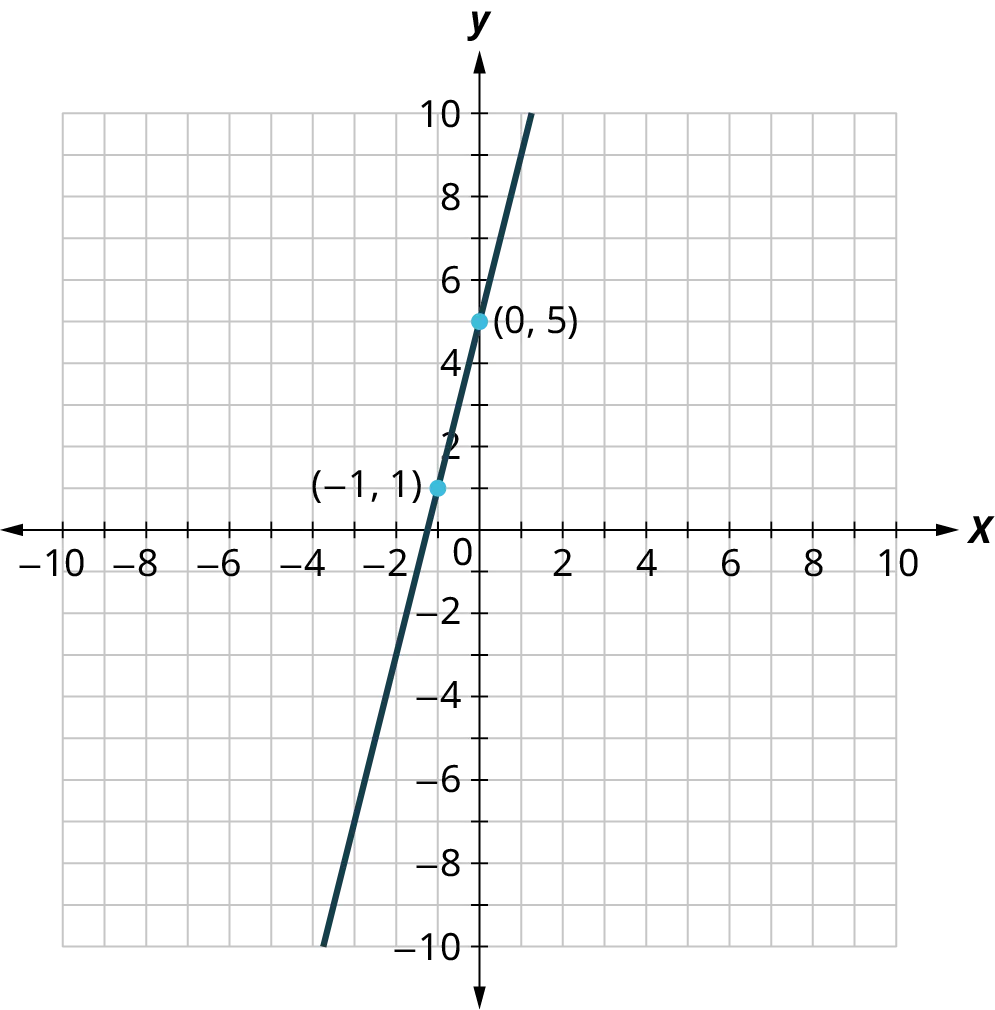64.
True or False. This graph has a slope of 5.
1. True
2. False
65.
True or False. This is the graph of the equation $y = {\text{ }}4x + 5$ .
1. True
2. False
66.
True or False. All vertical lines have a slope of zero.
1. True
2. False

### Section 5.8 Exercises

For the following exercises, find the $x$- and $y$-intercepts on the graph.
1 .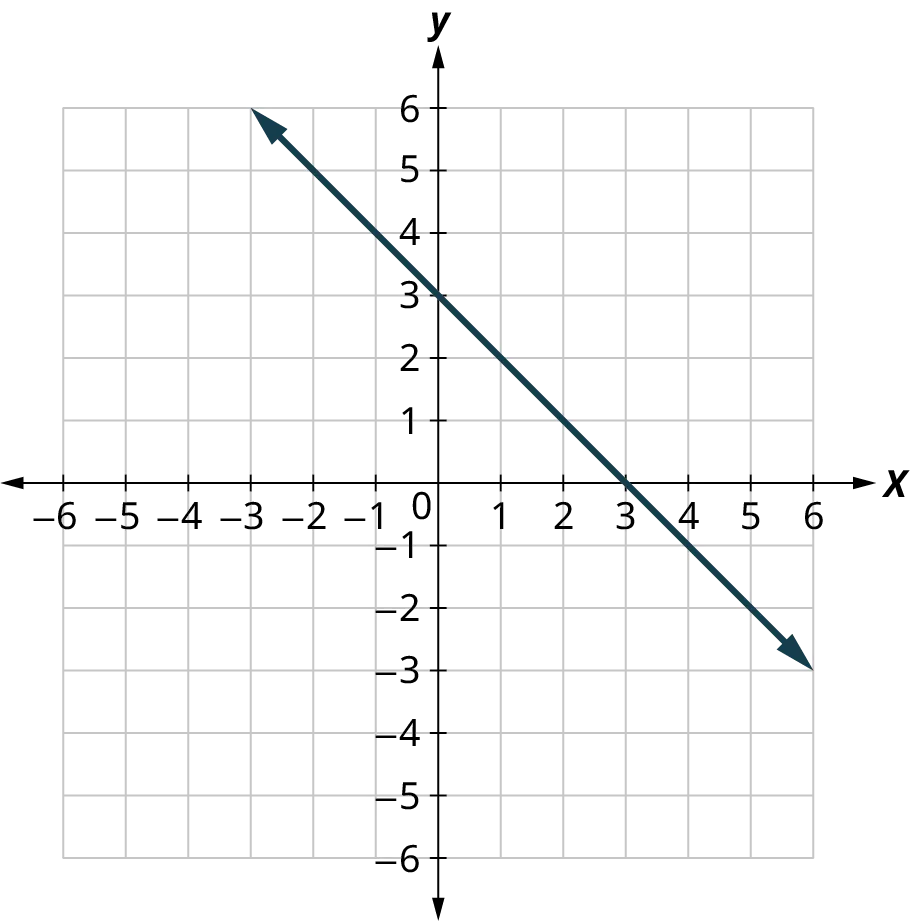2 .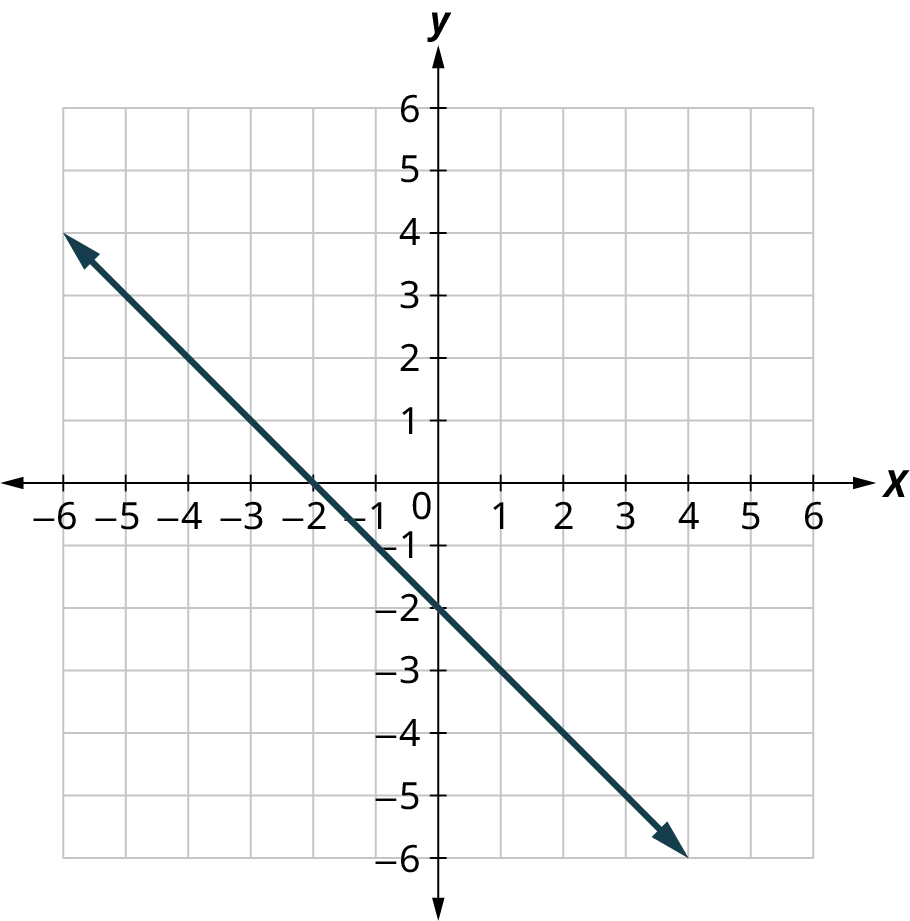3 .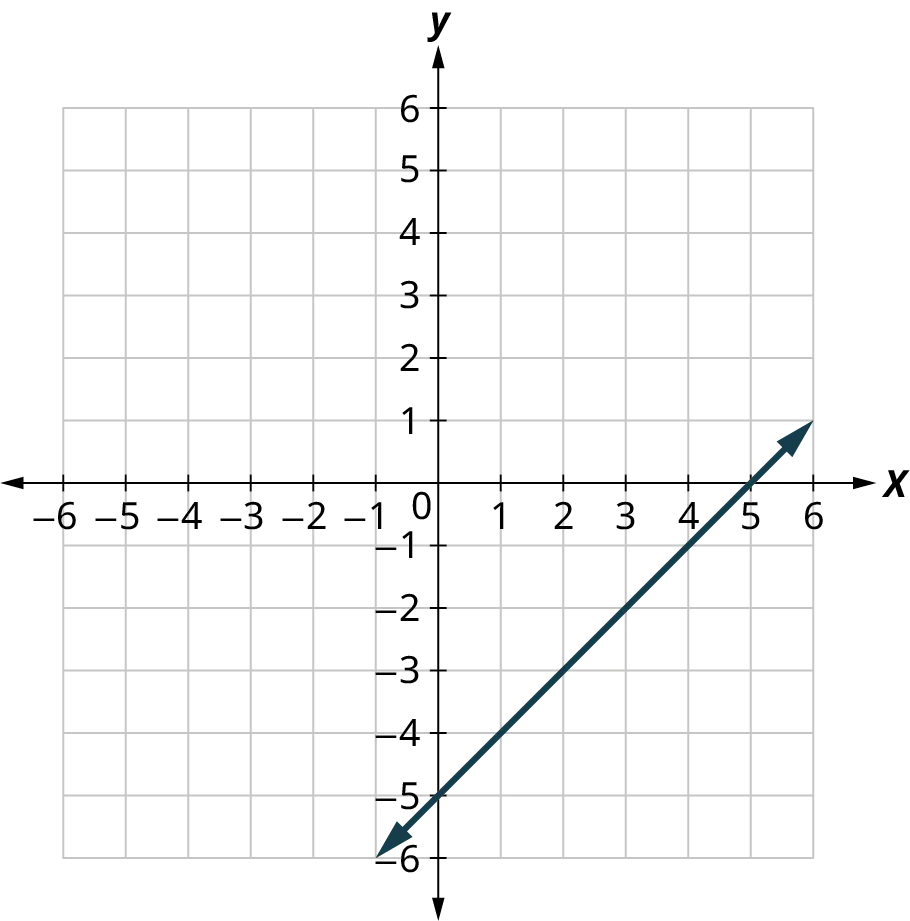4 .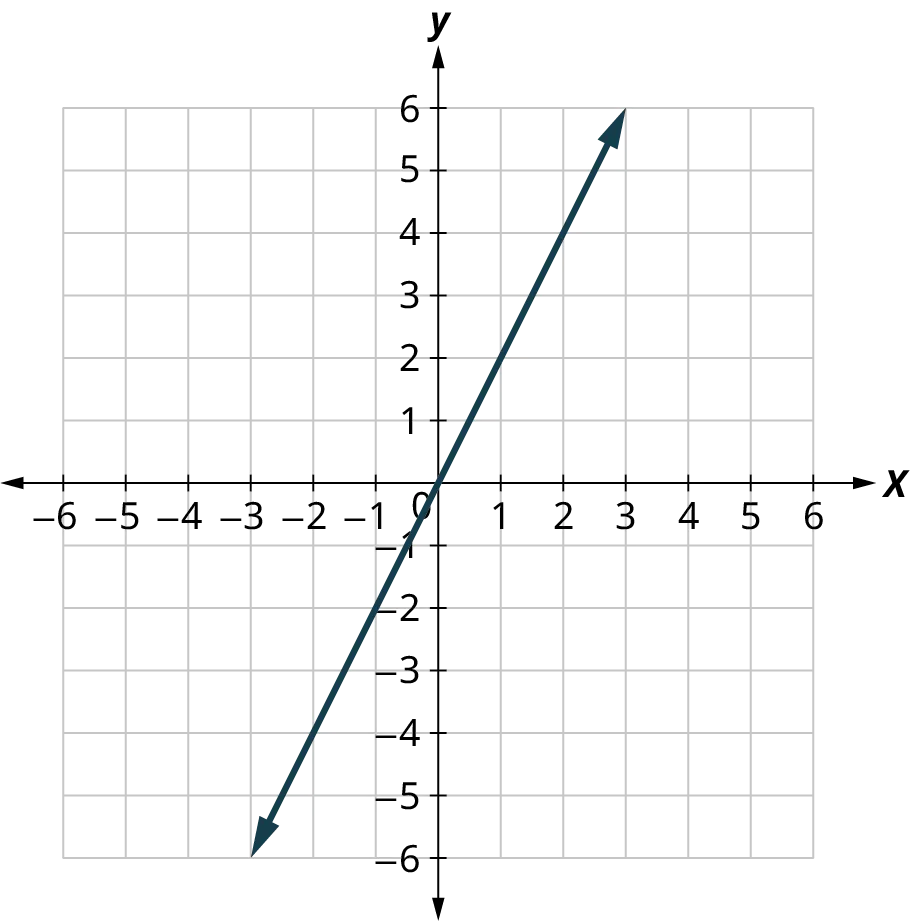For the following exercises, graph using the intercepts.
5 .
$- x + 4y = 8$
6 .
$x + y = - 3$
7 .
$4x + y = 4$
8 .
$3x - y = - 6$
9 .
$2x + 4y = 12$
10 .
$2x - 5y = - 20$
11 .
$y = - 2x$
12 .
$y = x$
For the following exercises, find the slope of the line.
13 .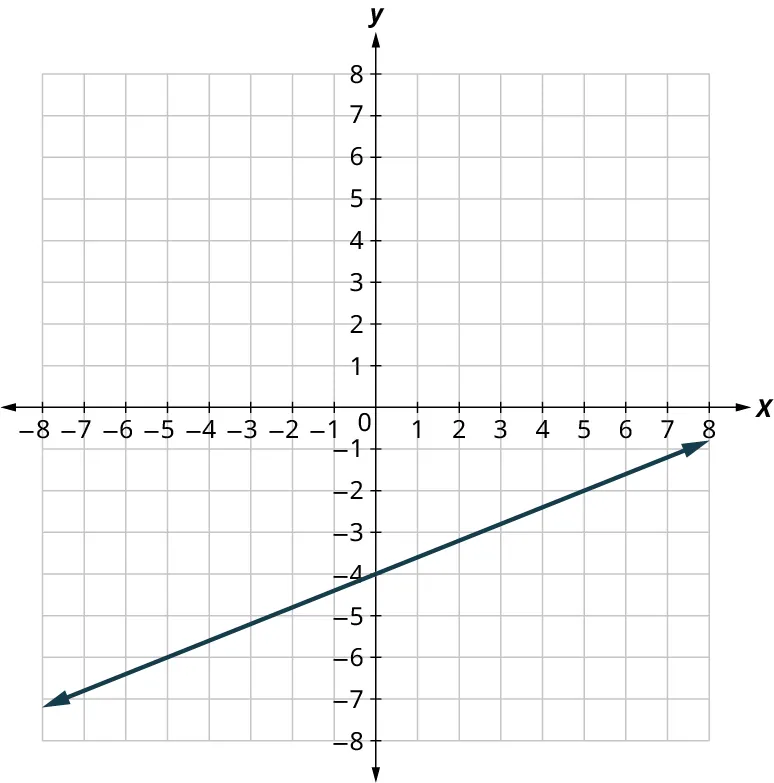14 .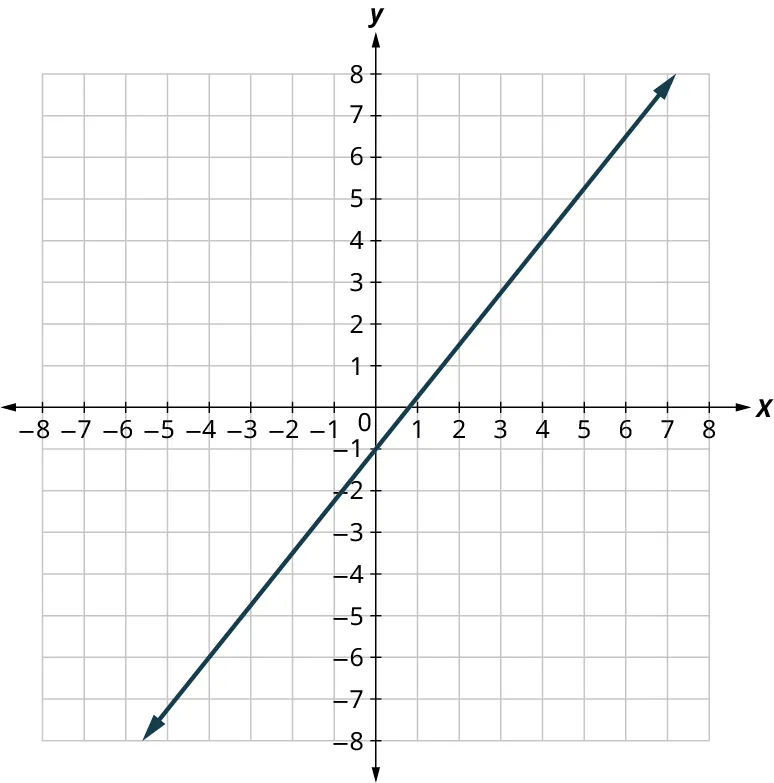15 .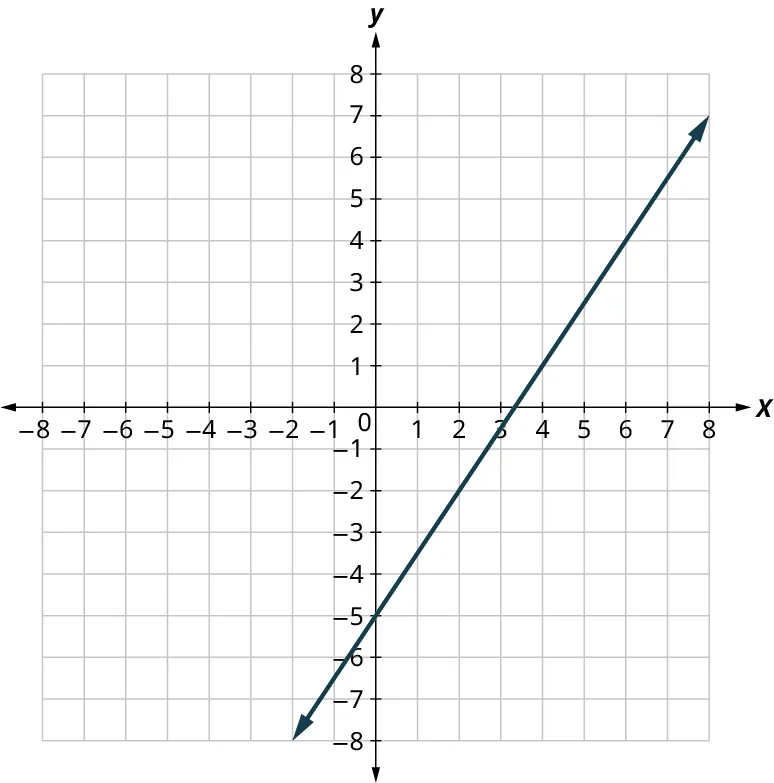16 .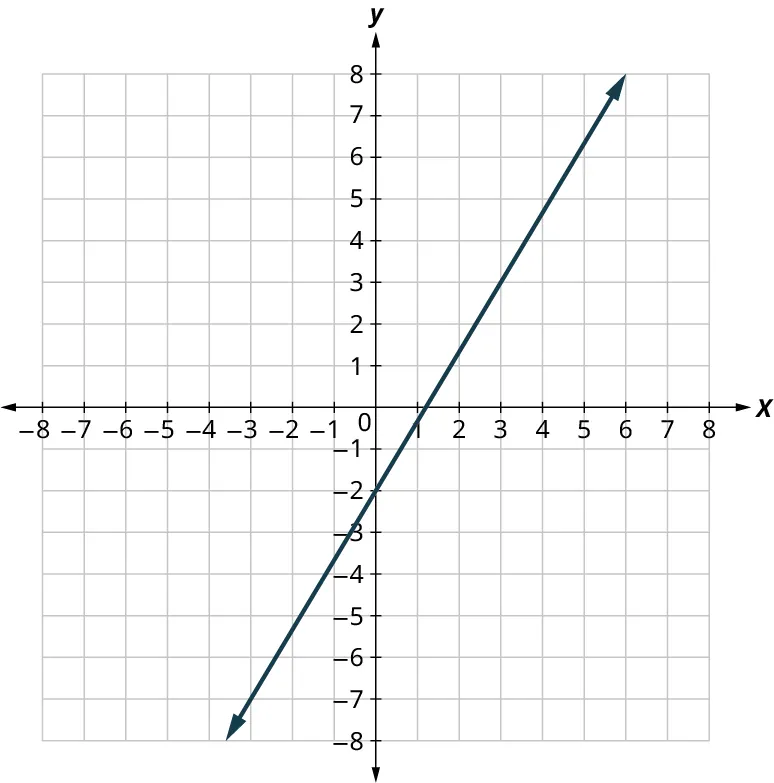17 .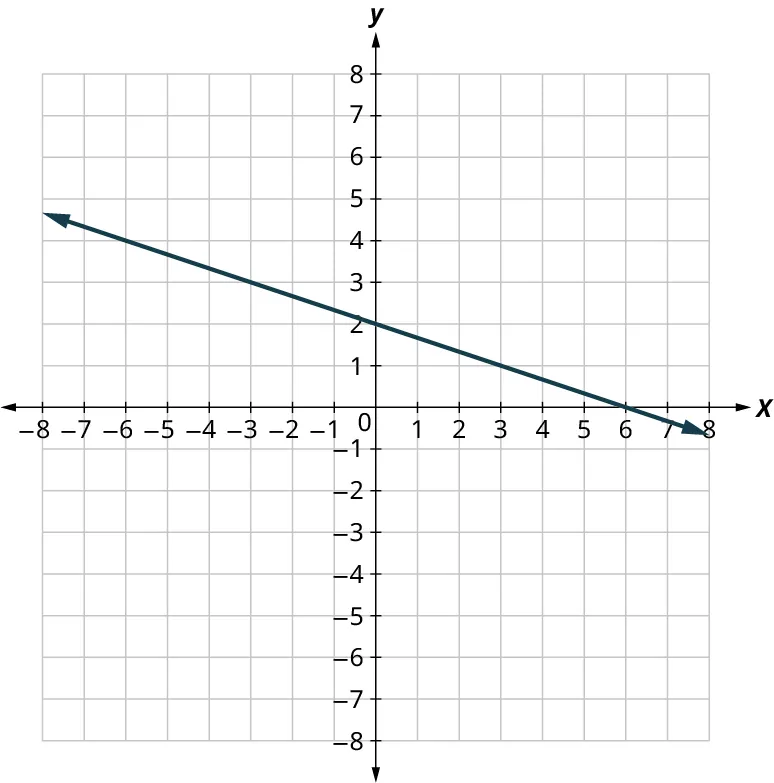18 .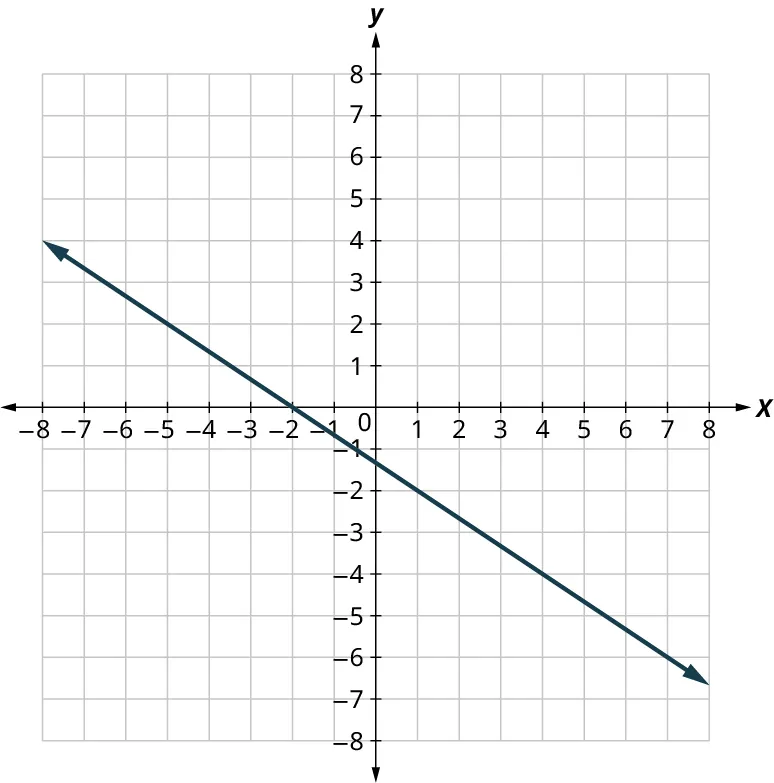19 .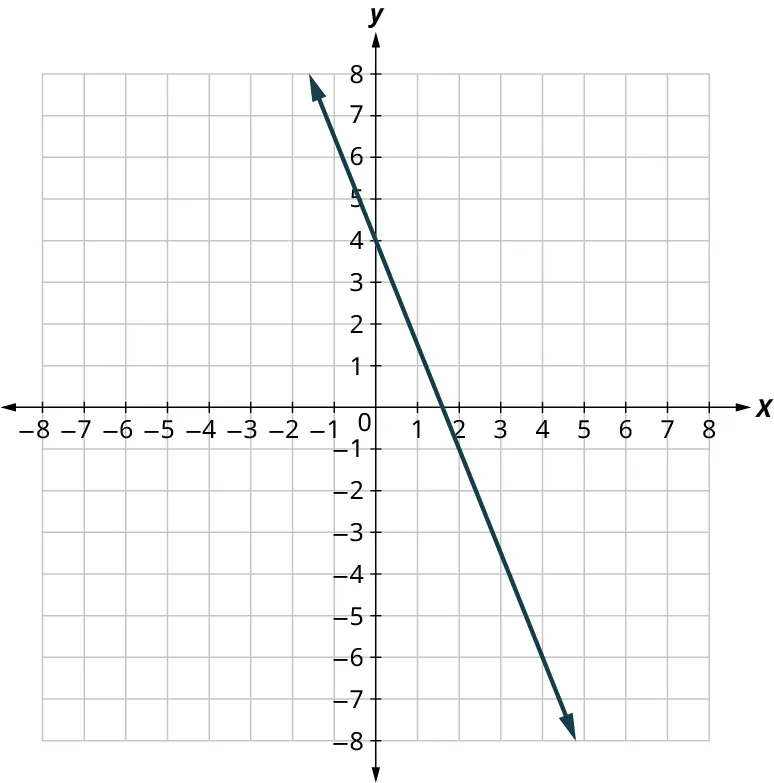20 .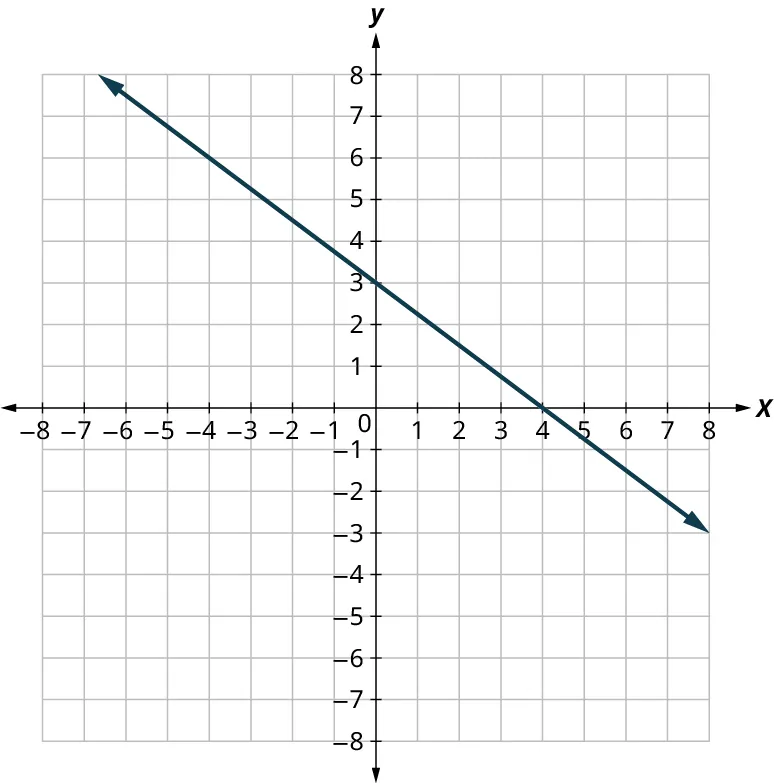For the following exercises, use the slope formula to find the slope of the line between each pair of points.
21 .
(2, 5), (4, 0)
22 .
(−3, 3), (4, −5)
23 .
(−1, −2), (2, 5)
24 .
(4, −5), (1, −2)
For the following exercises, identify the slope and $y$-intercept of each line.
25 .
$y = - 7x + 3$
26 .
$y = 4x - 10$
27 .
$3x + y = 5$
28 .
$4x + y = 8$
29 .
$6x + 4y = 12$
30 .
$8x + 3y = 12$
31 .
$5x - 2y = 6$
32 .
$7x - 3y = 9$
For the following exercises, graph the line of each equation using its slope and $y$-intercept.
33 .
$y = 3x - 1$
34 .
$y = 2x - 3$
35 .
$y = - x + 3$
36 .
$y = - x - 4$
37 .
$y = - 2x - 3$
38 .
$y = - 3x + 2$
39 .
$3x - 2y = 4$
40 .
$3x - 4y = 8$
For the following exercises, find the slope of each line and graph.
41 .
$y = 3$
42 .
$y = - 2$
43 .
$x = - 5$
44 .
$x = 4$
For the following exercises, graph and interpret applications of slope-intercept.
The equation $P = 31 + 1.75w$ models the relation between the amount of Tuyet’s monthly water bill payment, $P$, in dollars, and the number of units of water, $w$, used.
45 .
Find Tuyet’s payment for a month when 0 units of water are used.
46 .
Find Tuyet’s payment for a month when 12 units of water are used.
47 .
Interpret the slope and $P$-intercept of the equation.
48 .
Graph the equation.
For the following exercises, graph and interpret applications of slope-intercept.
Bruce drives his car for his job. The equation $R = 0.575m + 42$ models the relation between the amount in dollars, $R$, that he is reimbursed and the number of miles, $m$, he drives in one day.
49 .
Find the amount Bruce is reimbursed on a day when he drives 0 miles.
50 .
Find the amount Bruce is reimbursed on a day when he drives 220 miles.
51 .
Interpret the slope and $R$-intercept of the equation.
52 .
Graph the equation.
For the following exercises, graph and interpret applications of slope-intercept.
Cherie works in retail and her weekly salary includes commission for the amount she sells. The equation $S = 400 + 0.15c$ models the relation between her weekly salary, $S$, in dollars and the amount of her sales, $c$, in dollars.
53 .
Find Cherie’s salary for a week when her sales were $0. 54 . Find Cherie’s salary for a week when her sales were$3,600.
55 .
Interpret the slope and $S$-intercept of the equation.
56 .
Graph the equation.
For the following exercises, graph and interpret applications of slope-intercept.
Costa is planning a lunch banquet. The equation $C = 450 + 28g$ models the relation between the cost in dollars, $C$, of the banquet and the number of guests, $g$.
57 .
Find the cost if the number of guests is 40.
58 .
Find the cost if the number of guests is 80.
59 .
Interpret the slope and $C$-intercept of the equation.
60 .
Graph the equation.
Order a print copy

As an Amazon Associate we earn from qualifying purchases.